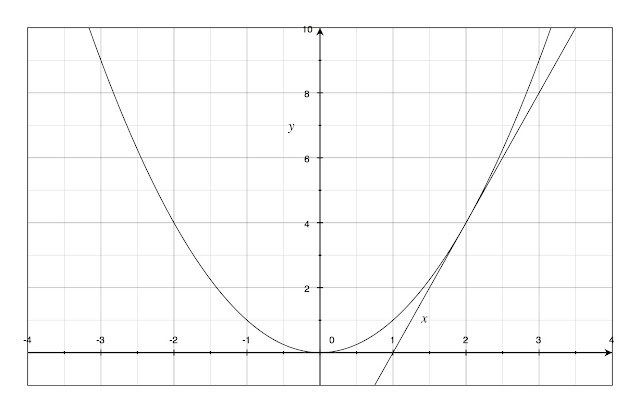# Using Limits to Find Tangents

Having discussed in several posts the concept of limits in mathematics, in this post, I am going to describe a more practical use for limits so that you can see how useful they can be.  To do this, I would like to discuss tangents to curves (not to be confused with the tangent trigonometric function!).  You are probably already familiar with the concept of a tangent line, but here is a more descriptive definition of it:  a tangent can be defined as a line (or a three dimensional plane) that touches a curve (or a surface) at a single point, but does not intersect it or cross it.

Our problem, though, is how to express this line (let's stay away from the 3D surfaces and planes for now!) in terms of the math that we already know.  We can always draw an approximation of a tangent line to a curve, but how can we precisely define that line and express it in terms of an equation?  This is where limits come in!

Let's consider a basic example.  Consider the parabola described by the function f(x) = x2.  How can we describe the line that is tangent to the curve when x = 2?Evaluating the tangent line to the parabola at the point (2,4)

Well, think about it this way.  How would you go about finding the equation of any other line?  It doesn't have to be a tangent or any other special line.  How would we find the equation of any line?  One of the first things that we'd need to know to write the equation of the line is the slope.  Now with that thought in mind, let's connect that to the concept of limits.

To find a slope of a line, we need two points and we calculate the slope m based on the differences in x and y values.  So, if we take any other point that lies on the curve, and we connect that point to the point where we want to evaluate the tangent line (here, at x=2), we would create a very rough approximation of our tangent line and can solve its slope knowing the coordinates of the two points.  (When we connect two points on a curve like this with a straight line, this line is referred to as a secant line.)  If we take points on the curve that are progressively closer and closer to our point of interest, the created secant lines more and more approximate the actual tangent line.  I've tried to demonstrate this in the following picture:Approximating the tangent slope by analyzing the slopes of secant lines

As you move along the curve from point A to B to C, the slope of the secant lines AP, BP, CP get closer and closer to the actual slope of the tangent at point P.  For good practice, try solving the equations of the lines for the 3 shown secants.  I have only plotted whole numbers, but you can better see the secant slopes approaching the tangent slope if you evaluate the lines formed by points even closer to P (try when x=1.9, then 1.99, then 1.999).  Once you can see what the slope of the tangent line will be, then it is only a matter of substituting that value, and the values of the ordered pair, into our favourite formula y=mx+b to solve for b, and then you can express the equation of the line in either standard form or point-slope form.  I have already done the work, and determined that the equation of the tangent line is y=4x-4.

So, hopefully you can see here the connection to limits!  As our extra points approach our point of interest, the slope of the secant approaches the slope of the tangent.  This is essentially what we do when we consider limits; that is, we are interested in what is happening as we get infinitely closer to our point of interest.  In this case, we can say that "the limit of m (slope), as x approaches 2, is 4."

With that, you can see that limits are not just some abstract mathematical concept designed to confuse students.  Limits actually can be useful, and in this case, we have seen that they can be used to determine the equation of a line at a single point!

Please leave a comment if you would like a little more explanation or pointers.  Also, I'd appreciate anyone's input about my graphs... I am using a new program to draw them, and while I think they look good, I am still learning how it works.  And of course, please click the +1 below if you found this post helpful!  Thanks!